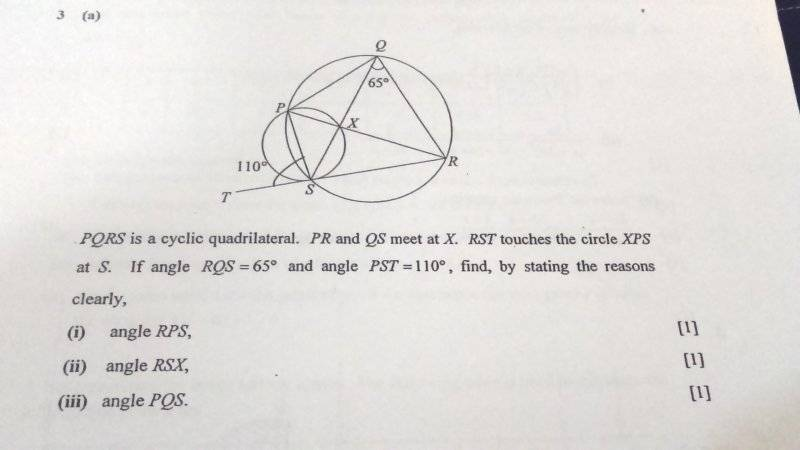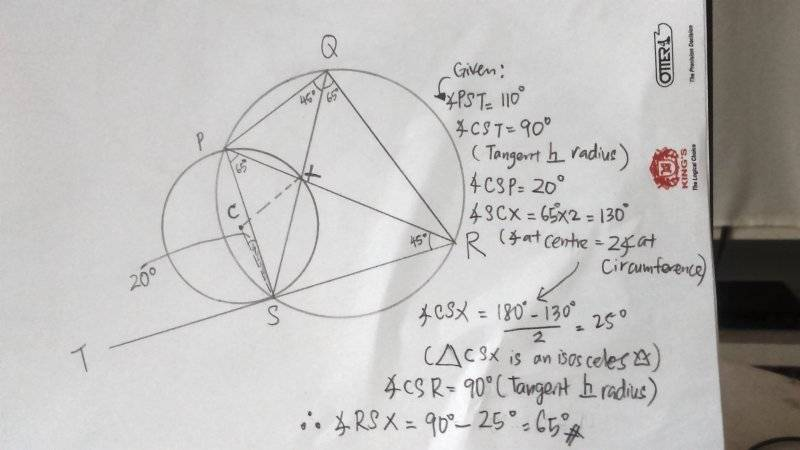# Geometrical properties of circle## Homework Statement

Dear Mentors/PF helpers,## The Attempt at a Solution

My solutions:
(i) angle RPS = 65 deg (angles in the same segment are equal)

(iii) angle PRS = 110 - 65 = 45 ( exterior angle of triangle PSR equal two interior angles)
angle PQS = angle PRS = 45 (angles in the same segment are equal)

mfb
Mentor
Hint: Introduce the point C as center of the circle XPS to use the "touching" information.mfb
Mentor
CSP should be PSC.

That is the same result I got.